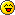Fibonacci Program

::  ::  ::Fibonacci Program

It does what the description says

Code:
`#include <iostream>using namespace std;int main(){int x,y,z;x=0;y=1;cout<<"The Fibonacci Sequence....\n\n";int i;cout<<x<<", ";cout<<y<<", ";for(i=0;i<30;i++){z=x+y;cout<<z<<", ";x=y;y=z;}system("pause");return 0;}`Pirate King

Posts : 559Really Allen?

Fibonacci Method 1:
Code:
`#include <iostream>#include <iomanip>using namespace std;int main(){   system("cls");   unsigned int numofnums;   cout << "how many numbers of the "        << "fibonacci sequence would"       << "you like to print: ";   cin >> numofnums;   cout.setf(ios_base::fixed, ios_base::floatfield);   double x, y, z;   x = 0;   y = 1;   if(numofnums <= 0)   {      return 0;   }   if(numofnums == 1)   {      cout << setprecision(0) << endl << x << endl;      return 0;   }   if((numofnums - 2) >= 0)   {      numofnums -= 2;   }   cout << setprecision(0) << x << endl << y << endl;   for(int i = 0; i < numofnums; i++)   {      z = x + y;      x = y;      y = z;      cout << setprecision(0) << z << endl;   }   return 0;}`

and

Fibonacci Method 2:
Code:
`#include <iostream>#include <iomanip>using namespace std;int main(){   system("cls");   unsigned long numofnums;   cout << "how many numbers of the "        << "fibonacci sequence would"       << "you like to print: ";   cin >> numofnums;   cout.setf(ios_base::fixed, ios_base::floatfield);   if(numofnums <= 0)   {      return 0;   }   if(numofnums == 1)   {      cout << endl << 0 << endl;      return 0;   }   for(unsigned long i = 0; i < numofnums; i++)   {      cout << setprecision(0) << fibonacci(i) << endl;   }   return 0;}double fibonacci(unsigned long num){   if(num>=2)return fibonacci(num-1)+fibonacci(num-2); //example of recursion, a function that calls itself   else if(num>0) return 1;   else return 0;}`Paul
Pickaxe

Posts : 611Re: Fibonacci Program

oooh so you're paul! lol i thought you were Brad.

now i recognize the "you fail"!Pirate King

Posts : 559Re: Fibonacci Program

One huge difference between my code and yours is that minw will print out truly gigantic numbers.Paul
Pickaxe

Posts : 611Re: Fibonacci Program

Why did you use cout 3 times instead of using /n ?Unchained
Mod

Posts : 448Re: Fibonacci Program

In my code?
The new line and the separate << makes no difference at all it prints all on one line.
In fact I don't even need the individual << for each line and it would still print on one line.Paul
Pickaxe

Posts : 611Re: Fibonacci Program